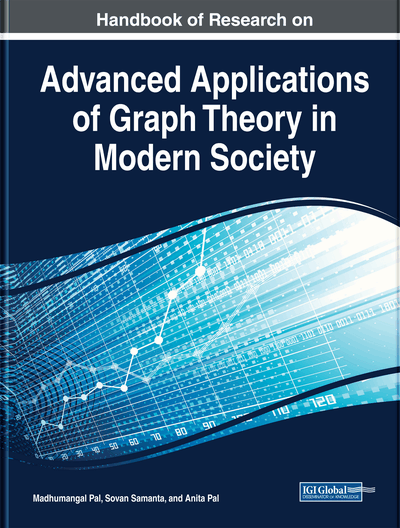# Energy of m-Polar Fuzzy Digraphs

Muhammad Akram (University of the Punjab, Pakistan), Danish Saleem (University of the Punjab, Pakistan) and Ganesh Ghorai (Vidyasagar University, India)
DOI: 10.4018/978-1-5225-9380-5.ch020
Available
\$37.50
No Current Special Offers

## Abstract

In this chapter, firstly some basic definitions like fuzzy graph, its adjacency matrix, eigenvalues, and its different types of energies are presented. Some upper bound and lower bound for the energy of this graph are also obtained. Then certain notions, including energy of m-polar fuzzy digraphs, Laplacian energy of m-polar fuzzy digraphs and signless Laplacian energy of m-polar fuzzy digraphs are presented. These concepts are illustrated with several example, and some of their properties are investigated.
Chapter Preview
Top

## Introduction

After the introduction of fuzzy sets by Zadeh (1965), fuzzy set theory has been applied in many research fields. Zhang (1994) presented the concept of bipolar fuzzy sets by depicting a positive degree of membership and negative degree of membership, which is an extension of fuzzy sets. This idea has been utilized in different ways, including preference modeling, multi-criteria decision inquiry and cooperative games. The positive degree of membership and negative degree of membership of bipolar fuzzy sets is lying in the interval [-1,1]. The degree of membership 0 of a component in a bipolar fuzzy set implies that the component is inapplicable to the property (K. M. Lee, 2000), the positive degree of membership (0,1] of a component argue that the component obviously satisfies the corresponding property and the negative degree of membership [-1,0) of a component argue that the component apparently satisfies the counter-property. Chen et al. (2014) introduced-polar fuzzy sets as generalization of bipolar fuzzy sets. He demonstrated that bipolar fuzzy sets and 2-polar fuzzy sets are cryptomorphic mathematical notions and that we can acquire briefly one from the corresponding one. The concept back of this is that “multipolar information” (not just bipolar information which correspond to two-valued logic) exists because the data for the real world problems is sometimes taken from n agents. For example, the exact degree of elecommunication safety of mankind is a point in() because different persons have been monitored different times. There are many other examples such as truth degrees of a logic formula which are based onlogic implication operators (), similarity degrees of two logic formulas which are based onlogic implication operators (), ordering results of a magazine, ordering results of a university, and inclusion degrees (accuracy measures, rough measures, approximation qualities, fuzziness measures, and decision preformation evaluations) of a rough set.

## Complete Chapter List

Search this Book:
Reset